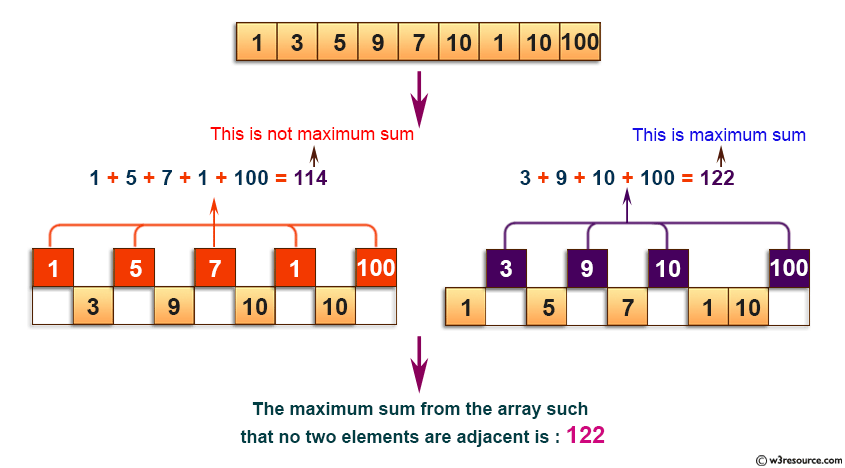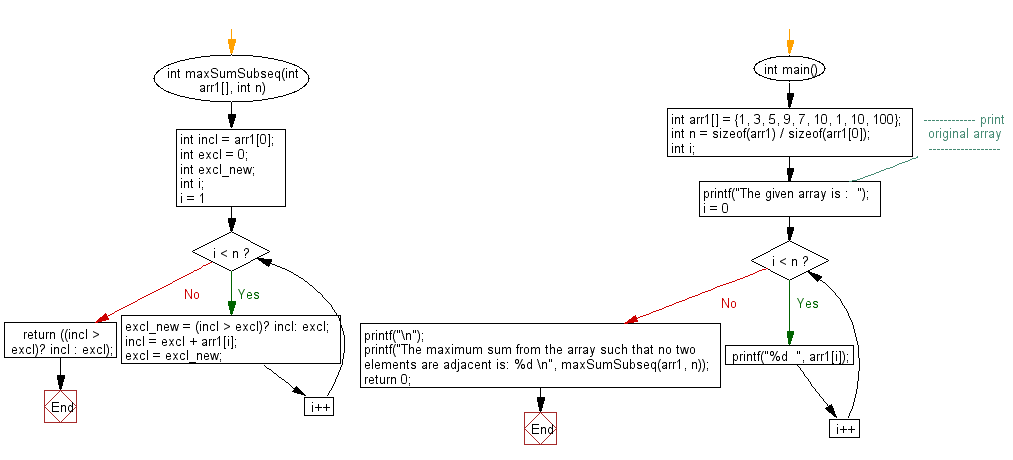﻿ C exercises: Return maximum sum such that no two elements are adjacent - w3resource# C Exercises: Return maximum sum such that no two elements are adjacent

## C Array: Exercise-68 with Solution

Write a program in C to return maximum sum such that no two elements are adjacent.

Pictorial Presentation:Sample Solution:

C Code:

``````#include <stdio.h>

int maxSumSubseq(int arr1[], int n)
{
int incl = arr1;
int excl = 0;
int excl_new;
int i;
for (i = 1; i < n; i++)
{
excl_new = (incl > excl)? incl: excl;

incl = excl + arr1[i];
excl = excl_new;
}
return ((incl > excl)? incl : excl);

}

int main()
{
int arr1[] = {1, 3, 5, 9, 7, 10, 1, 10, 100};
int n = sizeof(arr1) / sizeof(arr1);
int i;
//------------- print original array ------------------
printf("The given array is :  ");
for(i = 0; i < n; i++)
{
printf("%d  ", arr1[i]);
}
printf("\n");
//------------------------------------------------------
printf("The maximum sum from the array such that no two elements are adjacent is: %d \n", maxSumSubseq(arr1, n));
return 0;
}
```
```

Sample Output:

```The given array is :  1  3  5  9  7  10  1  10  100
The maximum sum from the array such that no two elements are adjacent is: 122
```

Flowchart:C Programming Code Editor:

Improve this sample solution and post your code through Disqus.

What is the difficulty level of this exercise?

﻿

## C Programming: Tips of the Day

Where is the C auto keyword used?

auto is a modifier like static. It defines the storage class of a variable. However, since the default for local variables is auto, you don't normally need to manually specify it.

Ref : https://bit.ly/3yzwC9r